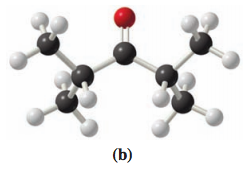# Problem: What is the molecular formula for each of the compounds given in Exercise 1.33?

###### FREE Expert Solution
86% (294 ratings)
###### Problem Details

What is the molecular formula for each of the compounds given in Exercise 1.33?What scientific concept do you need to know in order to solve this problem?

Our tutors have indicated that to solve this problem you will need to apply the Condensed Structural Formula concept. You can view video lessons to learn Condensed Structural Formula. Or if you need more Condensed Structural Formula practice, you can also practice Condensed Structural Formula practice problems.

What is the difficulty of this problem?

Our tutors rated the difficulty ofWhat is the molecular formula for each of the compounds give...as low difficulty.

How long does this problem take to solve?

Our expert Organic tutor, Jonathan took 1 minute and 3 seconds to solve this problem. You can follow their steps in the video explanation above.

What professor is this problem relevant for?

Based on our data, we think this problem is relevant for Professor Seitz & Labadie's class at UNH.

What textbook is this problem found in?

Our data indicates that this problem or a close variation was asked in Organic Chemistry - Solomons 10th Edition. You can also practice Organic Chemistry - Solomons 10th Edition practice problems.